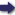(London :  Kegan Paul, Trench, Trübner & Co.,  1910.)

 Tools

## Search this bookPrev Page 42 Next``` 42 ALBERUNTS INDIA. Criticisms on the pas¬ sage from months, you get as quotient 976 days and a remainder of 104,064. The common divisor for this number and for the divisor is 384. Eeducing the fraction thereby, we get ■^,jyW;-uiTo days." Here, however, I suspect either the copyist or the translator, for Pulisa was too good a scholar to commit similar blunders. The matter is this :— Those days which are divided by the ctdhimdsa months are of necessity solctr days. The quotient con¬ tains wholes and fractions, as has been stated. Both denominator and numerator have as common divisor the number 24. Eeducing the fraction thereby, we get 4.3 3 6 6 6 , y 8 9 ■ If we apply this rule to the months, and reduce the number of adhimdsa months to fractions, we get 47,800,000 as denominator. A divisor common to both this denominator and its numerator is 16. Eeducing the fraction thereby, we get 2-,8"w,Wo- If we now multiply the number which Pulisa adopts as divisor by the just-mentioned common divisor, i.e. 384, we get the product 1,555,200,000, viz. solar days in a caturyitgct. But it is quite impossible that this number should, in this part of the calculation, be used as a divisor. If we want to base this method on the rules of Brahmagupta, dividing the universal solar months by the ctdhimdsct months, the result will be, according to the method employed by him, double the amount of the adhimdsct. Method for Further, a similar method may be used for the com- tation'^f^the putation of the iXnctrdtrct days. Wafra Write down the partial lunar days in two different places. In the one place, multiply the number by 50,663, and divide the product by 3,562,220. Sub¬ tract the quotient from the number in the other place, and divide the remainder by 63 without any fraction. Page 224. In the further very lengthy speculations of the I ```Prev Page 42 Next